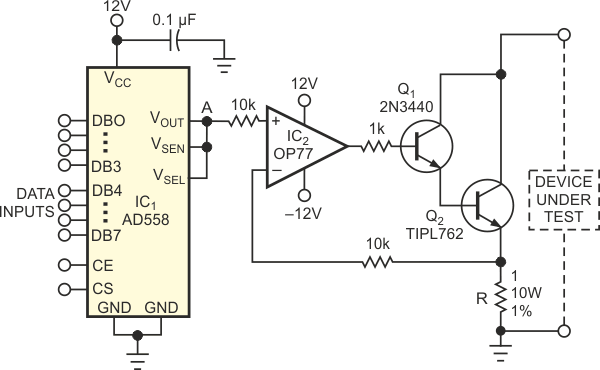# Variable load tests voltage sources

The circuit in Figure 1 serves as a variable, current-sink load for testing voltage sources. You use digital commands to set the load current of the device under test over a wide range, independently of the device under test's output voltage. The circuit comprises an AD558 DAC, IC1, which provides a reference voltage at Point A. Practically any type of DAC converter works well in this application. The AD558 is a single-supply type with an internal reference; these features simplify the design. IC1 generates an output voltage of 0 to 2.55 V (Table 1). The control inputs CE and CS in IC1 allow you to control the DAC from a microprocessor bus. If your application does not involve a data bus, connect CE and CS to ground to obtain direct access to the DAC's data inputs.

Table 1. DAC output voltage versus input code
 Digital input of IC1 Voltage at Point A (V) Binary Hexadecimal 0000 0000 00 0 0000 0001 01 0.01 0000 1111 0F 0.15 0001 0000 10 0.16 1000 0000 80 1.28 1111 1111 FF 2.55Figure 1. A simple circuit allows digital control of current, independent of voltage.

The second part of the circuit in Figure 1 consists of an op amp, IC2, driving transistors Q1 and Q2. IC2 compares the reference voltage at Point A with the voltage across resistor R. IC2’s output voltage controls Q1 and Q2 such that the voltage across R equals the reference voltage at point A. The voltage across R is proportional to the current from the device under test and is independent of the output voltage of the device under test. The value of R in Figure 1 is 1 Ω; thus, the circuit provides a sink current of 1 A when the voltage at point A is 1 V. With the values shown in Figure 1, you can control currents of 0 to 2.55 A over a device under test voltage range of 5 to 250 V. Be sure to limit the power dissipation in Q2 to 120 W.

## Materials on the topic

EDN